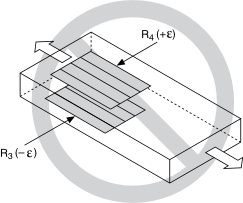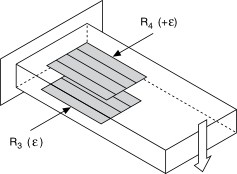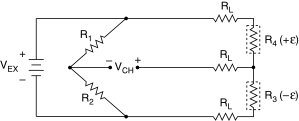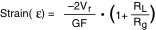# Half-Bridge Type II

## NI-DAQmx 18.6 Help

Edition Date: January 2019
Part Number: 370466AH-01
View Product InfoNI-DAQmx 18.6 HelpNI-DAQmx 19.0 HelpNI-DAQmx 19.1 HelpNI-DAQmx 19.5 Help

The half-bridge type II configuration only measures bending strain.The following figure shows how to position strain gage resistors in a bending configuration for the half-bridge type II.Half-bridge type II strain gage configurations have the following characteristics:

• Two active strain gage elements, one mounted in the direction of axial strain on the top side of the strain specimen and the other mounted in the direction of axial strain on the bottom side.
• Completion resistors which provide half-bridge completion.
• Sensitivity to bending strain.
• Rejection of axial strain.
• Compensation for temperature.
• Sensitivity at 1000 µε is ~ 1 mVout / VEX input.
Related concepts

## Half-Bridge Type II Circuit DiagramThe following symbols apply to the circuit diagram:
• R1 is the half-bridge completion resistor.
• R2 is the half-bridge completion resistor.
• R3 is the active strain gage element measuring compressive strain (–ε).
• R4 is the active strain gage resistor measuring tensile strain (+ε).
• VEX is the excitation voltage.
• RL is the lead resistance.
• VCH is the measured voltage.

The following equation converts voltage ratios to strain units for half-bridge type II configurations.where Vr is the voltage ratio that virtual channels use in the voltage-to-strain conversion equation, GF is the gage factor, RL is the lead resistance, and Rg is the nominal gage resistance.